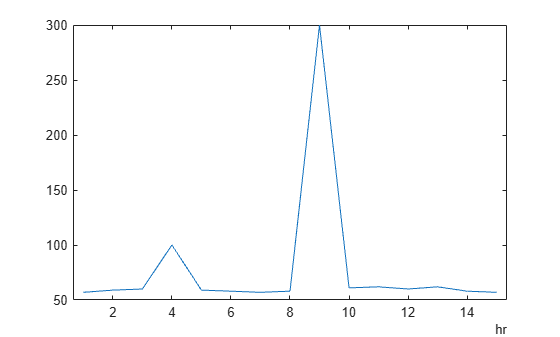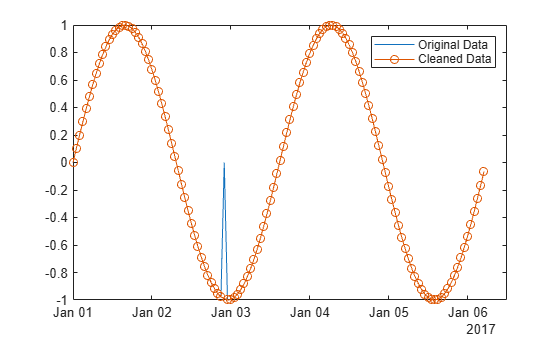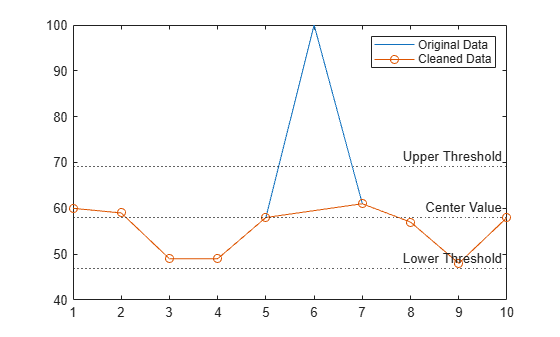# rmoutliers

Detect and remove outliers in data

## Syntax

``B = rmoutliers(A)``
``B = rmoutliers(A,method)``
``B = rmoutliers(A,"percentiles",threshold)``
``B = rmoutliers(A,movmethod,window)``
``B = rmoutliers(___,dim)``
``B = rmoutliers(___,Name,Value)``
``````[B,TFrm] = rmoutliers(___)``````
``````[B,TFrm,TFoutlier] = rmoutliers(___)``````
``````[B,TFrm,TFoutlier,L,U,C] = rmoutliers(___)``````

## Description

example

````B = rmoutliers(A)` detects and removes outliers from the data in `A`. If `A` is a matrix, then `rmoutliers` detects outliers in each column of `A` separately and removes the entire row.If `A` is a table or timetable, then `rmoutliers` detects outliers in each variable of `A` separately and removes the entire row. By default, an outlier is a value that is more than three scaled median absolute deviations (MAD) from the median.```

example

````B = rmoutliers(A,method)` specifies a method for detecting outliers. For example, `rmoutliers(A,"mean")` defines an outlier as an element of `A` more than three standard deviations from the mean.```
````B = rmoutliers(A,"percentiles",threshold)` defines outliers as points outside of the percentiles specified in `threshold`. The `threshold` argument is a two-element row vector containing the lower and upper percentile thresholds, such as ```[10 90]```.```

example

````B = rmoutliers(A,movmethod,window)` detects local outliers using a moving window mean or median with window length `window`. For example, `rmoutliers(A,"movmean",5)` defines outliers as elements more than three local standard deviations from the local mean within a five-element window.```

example

````B = rmoutliers(___,dim)` specifies the dimension of `A` for which to remove entries when an outlier is detected using any of the previous syntaxes. For example, `rmoutliers(A,2)` removes columns instead of rows for a matrix `A`.```

example

````B = rmoutliers(___,Name,Value)` specifies additional parameters for detecting and removing outliers using one or more name-value arguments. For example, `rmoutliers(A,"SamplePoints",t)` detects outliers in `A` relative to the corresponding elements of a time vector `t`.```

example

``````[B,TFrm] = rmoutliers(___)``` also returns a logical vector `TFrm` that indicates the rows or columns removed from `A`.```

example

``````[B,TFrm,TFoutlier] = rmoutliers(___)``` also returns a logical array `TFoutlier` that indicates the locations of the outliers removed from `A`.```

example

``````[B,TFrm,TFoutlier,L,U,C] = rmoutliers(___)``` also returns the lower threshold `L`, upper threshold `U`, and center value `C` used by the outlier detection method.```

## Examples

collapse all

Create a vector containing two outliers and remove them.

```A = [57 59 60 100 59 58 57 58 300 61 62 60 62 58 57]; B = rmoutliers(A)```
```B = 1×13 57 59 60 59 58 57 58 61 62 60 62 58 57 ```

Identify potential outliers in a timetable of data using the mean detection method, remove any outliers, and visualize the cleaned data.

Create a timetable of data, and visualize the data to detect potential outliers.

```T = hours(1:15); V = [57 59 60 100 59 58 57 58 300 61 62 60 62 58 57]; A = timetable(T',V'); plot(A.Time,A.Var1)```Remove outliers in the data, where an outlier is defined as a point more than three standard deviations from the mean.

`B = rmoutliers(A,"mean")`
```B=14×1 timetable Time Var1 _____ ____ 1 hr 57 2 hr 59 3 hr 60 4 hr 100 5 hr 59 6 hr 58 7 hr 57 8 hr 58 10 hr 61 11 hr 62 12 hr 60 13 hr 62 14 hr 58 15 hr 57 ```

In the same graph, plot the original data and the data with the outlier removed.

```hold on plot(B.Time,B.Var1,"o-") legend("Original Data","Cleaned Data")```Use a moving median to detect and remove local outliers from a sine wave that corresponds to a time vector.

Create a vector of data containing a local outlier.

```x = -2*pi:0.1:2*pi; A = sin(x); A(47) = 0;```

Create a time vector that corresponds to the data in `A`.

`t = datetime(2017,1,1,0,0,0) + hours(0:length(x)-1);`

Define outliers as points more than three local scaled MAD from the local median within a sliding window. Find the locations of the outliers in `A` relative to the points in `t` with a window size of 5 hours, and remove them.

`[B,TFrm] = rmoutliers(A,"movmedian",hours(5),"SamplePoints",t);`

Plot the original data and the data with the outlier removed.

```plot(t,A) hold on plot(t(~TFrm),B,"o-") legend("Original Data","Cleaned Data")```Remove the outliers from a matrix of data, and examine the removed columns and outliers.

Create a matrix containing two outliers.

```A = magic(5); A(4,4) = 200; A(5,5) = 300; A```
```A = 5×5 17 24 1 8 15 23 5 7 14 16 4 6 13 20 22 10 12 19 200 3 11 18 25 2 300 ```

Remove the columns containing outliers by specifying the dimension for removal as 2. Return a logical output vector `TFrm` to identify which columns of `A` were removed, and return a logical output array `TFoutlier` to identify the locations of the outliers in `A`.

`[B,TFrm,TFoutlier] = rmoutliers(A,2)`
```B = 5×3 17 24 1 23 5 7 4 6 13 10 12 19 11 18 25 ```
```TFrm = 1x5 logical array 0 0 0 1 1 ```
```TFoutlier = 5x5 logical array 0 0 0 0 0 0 0 0 0 0 0 0 0 0 0 0 0 0 1 0 0 0 0 0 1 ```

Find the values in the removed columns of `A`.

`rmCol = A(:,TFrm)`
```rmCol = 5×2 8 15 14 16 20 22 200 3 2 300 ```

Find the values of the outliers in `A`.

`rmVal = A(TFoutlier)`
```rmVal = 2×1 200 300 ```

Create a vector containing two outliers and detect their locations.

```A = [57 59 60 100 59 58 57 58 300 61 62 60 62 58 57]; detect = isoutlier(A)```
```detect = 1x15 logical array 0 0 0 1 0 0 0 0 1 0 0 0 0 0 0 ```

Remove the outliers. Instead of using a detection method, provide the outlier locations detected by `isoutlier`.

`B = rmoutliers(A,"OutlierLocations",detect)`
```B = 1×13 57 59 60 59 58 57 58 61 62 60 62 58 57 ```

Remove an outlier from a vector of data and visualize the cleaned data.

Create a vector of data containing an outlier.

`A = [60 59 49 49 58 100 61 57 48 58];`

Remove the outlier using the default detection method `"median"`.

`[B,TFrm,TFoutlier,L,U,C] = rmoutliers(A);`

Plot the original data, the data with outliers removed, and the thresholds and center value determined by the detection method. The center value is the median of the data, and the upper and lower thresholds are three scaled MAD above and below the median.

```plot(A) hold on plot(find(~TFrm),B,"o-") yline([L U C],":",["Lower Threshold","Upper Threshold","Center Value"]) legend("Original Data","Cleaned Data")```## Input Arguments

collapse all

Input data, specified as a vector, matrix, table, or timetable.

• If `A` is a table, then its variables must be of type `double` or `single`, or you can use the `DataVariables` argument to list `double` or `single` variables explicitly. Specifying variables is useful when you are working with a table that contains variables with data types other than `double` or `single`.

• If `A` is a timetable, then `rmoutliers` operates only on the table elements. If row times are used as sample points, then they must be unique and listed in ascending order.

Data Types: `double` | `single` | `table` | `timetable`

Method for detecting outliers, specified as one of these values.

MethodDescription
`"median"`Outliers are defined as elements more than three scaled MAD from the median. The scaled MAD is defined as `c*median(abs(A-median(A)))`, where `c=-1/(sqrt(2)*erfcinv(3/2))`.
`"mean"`Outliers are defined as elements more than three standard deviations from the mean. This method is faster but less robust than `"median"`.
`"quartiles"`Outliers are defined as elements more than 1.5 interquartile ranges above the upper quartile (75 percent) or below the lower quartile (25 percent). This method is useful when the data in `A` is not normally distributed.
`"grubbs"`Outliers are detected using Grubbs’ test for outliers, which removes one outlier per iteration based on hypothesis testing. This method assumes that the data in `A` is normally distributed.
`"gesd"`Outliers are detected using the generalized extreme Studentized deviate test for outliers. This iterative method is similar to `"grubbs"` but can perform better when there are multiple outliers masking each other.

Percentile thresholds, specified as a two-element row vector whose elements are in the interval [0, 100]. The first element indicates the lower percentile threshold, and the second element indicates the upper percentile threshold. The first element of `threshold` must be less than the second element.

For example, a threshold of `[10 90]` defines outliers as points below the 10th percentile and above the 90th percentile.

Moving method for detecting outliers, specified as one of these values.

MethodDescription
`"movmedian"`Outliers are defined as elements more than three local scaled MAD from the local median over a window length specified by `window`. This method is also known as a Hampel filter.
`"movmean"`Outliers are defined as elements more than three local standard deviations from the local mean over a window length specified by `window`.

Window length, specified as a positive integer scalar, a two-element vector of positive integers, a positive duration scalar, or a two-element vector of positive durations.

When `window` is a positive integer scalar, the window is centered about the current element and contains `window-1` neighboring elements. If `window` is even, then the window is centered about the current and previous elements.

When `window` is a two-element vector of positive integers `[b f]`, the window contains the current element, `b` elements backward, and `f` elements forward.

When `A` is a timetable or `SamplePoints` is specified as a `datetime` or `duration` vector, `window` must be of type `duration`, and the windows are computed relative to the sample points.

Dimension for removal, specified as 1 or 2. By default, `rmoutliers` removes each row with a detected outlier. To remove each matrix column or table variable with a detected outlier, specify a dimension of 2.

### Name-Value Arguments

Specify optional pairs of arguments as `Name1=Value1,...,NameN=ValueN`, where `Name` is the argument name and `Value` is the corresponding value. Name-value arguments must appear after other arguments, but the order of the pairs does not matter.

Example: `rmoutliers(A,ThresholdFactor=4)`

Before R2021a, use commas to separate each name and value, and enclose `Name` in quotes.

Example: `rmoutliers(A,"ThresholdFactor",4)`

Data Options

collapse all

Sample points, specified as either a vector of sample point values or one of the options in the following table when the input data is a table. The sample points represent the x-axis locations of the data, and must be sorted and contain unique elements. Sample points do not need to be uniformly sampled. The vector `[1 2 3 ...]` is the default.

When the input data is a table, you can specify the sample points as a table variable using one of these options.

Option for Table InputDescriptionExamples
Variable name

A character vector or scalar string specifying a single table variable name

`'Var1'`

`"Var1"`

Scalar variable index

A scalar table variable index

`3`

Logical vector

A logical vector whose elements each correspond to a table variable, where `true` specifies the corresponding variable as the sample points, and all other elements are `false`

`[true false false]`

Function handle

A function handle that takes a table variable as input and returns a logical scalar, which must be `true` for only one table variable

`@isnumeric`

`vartype` subscript

A table subscript generated by the `vartype` function that returns a subscript for only one variable

`vartype('numeric')`

Note

This name-value argument is not supported when the input data is a `timetable`. Timetables use the vector of row times as the sample points. To use different sample points, you must edit the timetable so that the row times contain the desired sample points.

Moving windows are defined relative to the sample points. For example, if `t` is a vector of times corresponding to the input data, then `rmoutliers(rand(1,10),"movmean",3,"SamplePoints",t)` has a window that represents the time interval between `t(i)-1.5` and `t(i)+1.5`.

When the sample points vector has data type `datetime` or `duration`, then the moving window length must have type `duration`.

Example: `rmoutliers(A,"SamplePoints",0:0.1:10)`

Example: `rmoutliers(T,"SamplePoints","Var1")`

Data Types: `single` | `double` | `datetime` | `duration`

Table variables to operate on, specified as one of the options in this table. The `DataVariables` value indicates which variables of the input table to examine for outliers. The data type associated with the indicated variables must be `double` or `single`.

Other variables in the table not specified by `DataVariables` pass through to the output without being examined for outliers.

When operating on the rows of `A`, `rmoutliers` removes any row that has outliers in the columns corresponding to the variables specified. When operating on the columns of `A`, `rmoutliers` removes the specified variables from the table.

OptionDescriptionExamples
Variable name

A character vector or string scalar specifying a single table variable name

`'Var1'`

`"Var1"`

Vector of variable names

A cell array of character vectors or string array, where each element is a table variable name

`{'Var1' 'Var2'}`

`["Var1" "Var2"]`

Scalar or vector of variable indices

A scalar or vector of table variable indices

`1`

`[1 3 5]`

Logical vector

A logical vector whose elements each correspond to a table variable, where `true` includes the corresponding variable and `false` excludes it

`[true false true]`

Function handle

A function handle that takes a table variable as input and returns a logical scalar

`@isnumeric`

`vartype` subscript

A table subscript generated by the `vartype` function

`vartype("numeric")`

Example: ```rmoutliers(T,"DataVariables",["Var1" "Var2" "Var4"])```

Outlier Detection Options

collapse all

Detection threshold factor, specified as a nonnegative scalar.

For methods `"median"` and `"movmedian"`, the detection threshold factor replaces the number of scaled MAD, which is 3 by default.

For methods `"mean"` and `"movmean"`, the detection threshold factor replaces the number of standard deviations from the mean, which is 3 by default.

For methods `"grubbs"` and `"gesd"`, the detection threshold factor is a scalar ranging from 0 to 1. Values close to 0 result in a smaller number of outliers, and values close to 1 result in a larger number of outliers. The default detection threshold factor is 0.05.

For the `"quartiles"` method, the detection threshold factor replaces the number of interquartile ranges, which is 1.5 by default.

This name-value pair is not supported when the specified method is `"percentiles"`.

Known outlier indicator, specified as a logical vector or matrix of the same size as `A`. The known outlier indicator elements can be a numeric or logical 1 (`true`) to indicate an outlier in the corresponding location of `A` or 0 (`false`) to indicate a nonoutlier. When you specify `OutlierLocations`, `rmoutliers` does not use an outlier detection method. Instead, it uses the elements of the known outlier indicator to define outliers. The output `TFoutlier` contains the same logical vector or matrix.

You cannot specify the `OutlierLocations` name-value argument if you specify `method`.

Data Types: `logical`

Maximum outliers detected by GESD, specified as a positive integer scalar. The `MaxNumOutliers` value specifies the maximum number of outliers that are detected by the `"gesd"` method. For example, `rmoutliers(A,"gesd","MaxNumOutliers",5)` detects no more than five outliers.

The default value for `MaxNumOutliers` is the integer nearest to 10 percent of the number of elements in `A`. Setting a larger value for the maximum number of outliers makes it more likely that all outliers are detected but at the cost of reduced computational efficiency.

The `"gesd"` method assumes the nonoutlier input data is sampled from an approximate normal distribution. When the data is not sampled in this way, the number of detected outliers might exceed the `MaxNumOutliers` value.

Minimum outliers required for removal, specified as a positive integer scalar. The `MinNumOutliers` value specifies the minimum number of outliers required to remove a row or column. For example, `rmoutliers(A,"MinNumOutliers",3)` removes a row of a matrix `A` when there are 3 or more outliers detected in that row.

## Output Arguments

collapse all

Data with outliers removed, returned as a vector, matrix, table, or timetable. The size of `B` depends on the number of removed rows or columns.

Removed data indicator, returned as a logical vector. Elements with a value of 1 (`true`) correspond to rows or columns of `A` that were removed. Elements with a value of 0 (`false`) correspond to unchanged rows or columns. The orientation and size of `TFrm` depend on `A` and the dimension of operation.

Data Types: `logical`

Outlier indicator, returned as a logical vector or matrix. Elements with a value of 1 (`true`) correspond to the location of outliers in `A`. Elements with a value of 0 (`false`) correspond to nonoutliers.

`TFoutlier` is the same size as `A`.

Data Types: `logical`

Lower threshold used by the outlier detection method, returned as a scalar, vector, matrix, table, or timetable. For example, the lower threshold value of the default outlier detection method is three scaled MAD below the median of the input data.

If `method` is used for outlier detection, then `L` has the same size as `A` in all dimensions except for the operating dimension where the length is 1. If `movmethod` is used, then `L` has the same size as `A`.

Upper threshold used by the outlier detection method, returned as a scalar, vector, matrix, table, or timetable. For example, the upper threshold value of the default outlier detection method is three scaled MAD above the median of the input data.

If `method` is used for outlier detection, then `U` has the same size as `A` in all dimensions except for the operating dimension where the length is 1. If `movmethod` is used, then `U` has the same size as `A`.

Center value used by the outlier detection method, returned as a scalar, vector, matrix, table, or timetable. For example, the center value of the default outlier detection method is the median of the input data.

If `method` is used for outlier detection, then `C` has the same size as `A` in all dimensions except for the operating dimension where the length is 1. If `movmethod` is used, then `C` has the same size as `A`.

## Version History

Introduced in R2018b

expand all